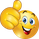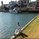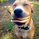# Indicators: Constance Brown Composite Index & RSI+Avgs

16310 vues
16310
I am a big fan of Constance Brown. Her book "Technical Analysis for Trading Professionals" is an absolute classic (get the 2nd edition).

I have included here 2 of the indicators she uses in all her charts.

Composite Index
----------------------------------------
This is a formula Ms Brown developed (Cardwell may not agree!) to identify divergence failures with in the RSI . This also highlights the horizontal support levels with in the indicator area.

This index removes the normalization range restrictions in RSI . This means it is not bound with in 0-100 range. Also, this has embedded momentum calculation in it.

The fine nuances of this indicator are not documented well enough, if you find some good documentation, do let me know. Always use this with RSI (like the next one).

RSI+Avgs
----------------------------------------
This is plain 14 period RSI with a 9-period EMA and 45-period SMA overlaid.
```//
// @author LazyBear
// Code from book "Breakthroughs in Technical Analysis" pg 95.
//
// If you use this code in its orignal/modified form, do drop me a note.
//
study(title="Constance Brown Composite Index [LazyBear]", shorttitle="CBIDX_LB")
src = close
rsi_length=input(14, title="RSI Length")
rsi_mom_length=input(9, title="RSI Momentum Length")
rsi_ma_length=input(3, title="RSI MA Length")
ma_length=input(3, title="SMA Length")
fastLength=input(13)
slowLength=input(33)

r=rsi(src, rsi_length)
rsidelta = mom(r, rsi_mom_length)
rsisma = sma(rsi(src, rsi_ma_length), ma_length)
s=rsidelta+rsisma

plot(s, color=red, linewidth=2)
plot(sma(s, fastLength), color=green)
plot(sma(s, slowLength), color=orange)
```
List of my free indicators: http://bit.ly/1LQaPK8
List of my indicators at Appstore: http://blog.tradingview.com/?p=970

## Commentaireshenjiro
PRO is not needed for using these free indicators.

Use this guide to import / use custom indicators published (PDF):

Here's a list of all my indicators. You can use the same guide above to import/use any of these.

RépondreI wanted to test these indicators but I cant see the "Make It Mine" button. Anyone knows why? What am I missing?
RépondreSir, could u please do similar work of overlaying EMA9 and SMA 26 on a Ultimate oscillator. I m not a programmer. Thanks
RépondreIt may be because I'm just too tired, but I'm not finding the code of the RSI w/MAs... I copied and pasted the Composite code, and it works fine, but where is the RSI w/MAs code? Sorry if I'm being dense...
Répondredenovo
Use this guide to import / use RSI+MAs. Note that this PDF shows the old UI, the "Share" button is the last button on the left side in the current UI.